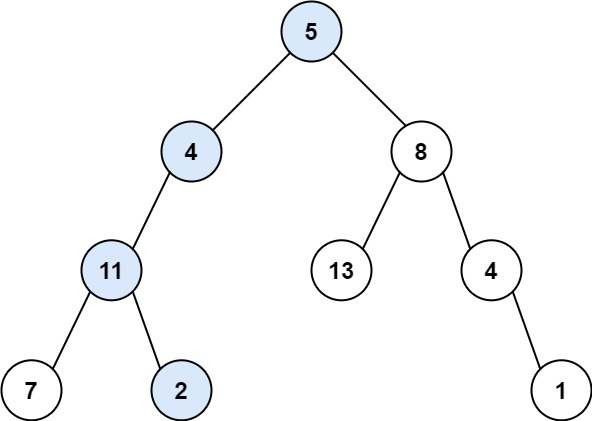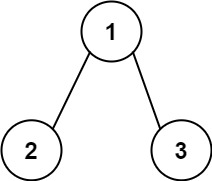| English | 简体中文 |

# 112. Path Sum

## Description

Given the root of a binary tree and an integer targetSum, return true if the tree has a root-to-leaf path such that adding up all the values along the path equals targetSum.

A leaf is a node with no children.

Example 1:Input: root = [5,4,8,11,null,13,4,7,2,null,null,null,1], targetSum = 22
Output: true


Example 2:Input: root = [1,2,3], targetSum = 5
Output: false


Example 3:

Input: root = [1,2], targetSum = 0
Output: false


Constraints:

• The number of nodes in the tree is in the range [0, 5000].
• -1000 <= Node.val <= 1000
• -1000 <= targetSum <= 1000